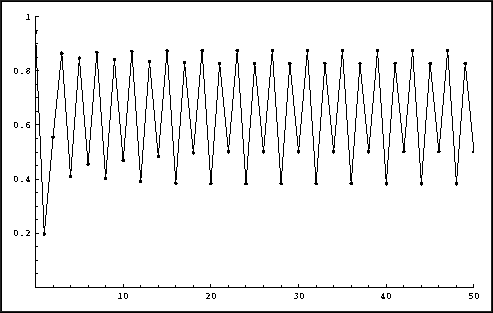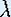Up: Iteration

# One-Dimensional Dynamical Systems

## Part 3: Iteration

#### Iterates versus time

The most intuitive way of vizualizing an orbit is by plotting the iterates versus time. Such time series were also produced in Part 2. Typically, measurements in experiments form such a time series. A lot of theory in dynamical systems is developed for constructing a model, i.e. an expression for the underlying function, out of the measurements.Plotting iterates versus time for the Logistic map.

Iterates versus time can be plotted with the following software. If you are using a Macintosh computer, follow the instructions for the software Chaos and Dynamics. On the other machines, you should follow the instructions for using Mathematica.

• Use the Java Applet Iterates versus time for the Logistic map to see how the plot changes asvaries. Describe what you see.

Note that always the iterates of x0 = 0.5 are taken. Can you find values for x0 (0) for which the orbit behaves differently from x0 = 0.5?

Up: IterationThe Geometry Center Home Page

Written by Hinke Osinga